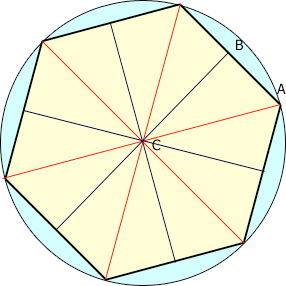SEARCH HOMEMath Central Quandaries & QueriesQuestion from jim, a student: A regular hexagon with an area of 24√3 is inscribed in a circle. What is the area of the circle?Hi Jim,

Divide the hexagon into 12 congruent right triangles as in the diagram.What is the area of te triangle ABC?

What is the measure of the angle BCA?

Can you now determine the radius of the circle, |CA|?

PennyMath Central is supported by the University of Regina and The Pacific Institute for the Mathematical Sciences.